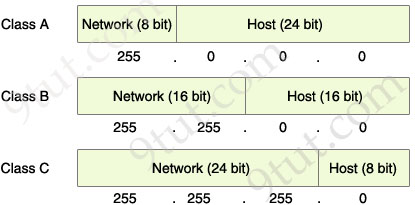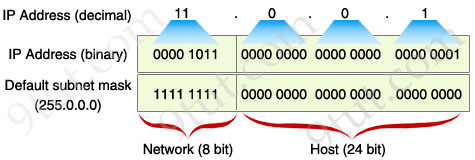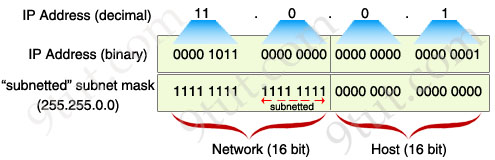# 04 – Subnetting 16,990 views

Posted by Mo7sin in On 24/01/2012 at 10:52 PM

The table below summarizes the possible network numbers, the total number of each type, and the number of hosts in each Class A, B, and C network.

 Default subnet mask Range Class A 255.0.0.0 (/8) 1.0.0.0 – 126.255.255.255 Class B 255.255.0.0 (/16) 128.0.0.0 – 191.255.255.255 Class C 255.255.255.0 (/24) 192.0.0.0 – 223.255.255.255

Table 1 – Default subnet mask & range of each class

Class A addresses begin with a 0 bit. Therefore, all addresses from 1.0.0.0 to 126.255.255.255 belong to class A (1=0000 0001; 126 = 0111 1110).
The 0.0.0.0 address is reserved for default routing and the 127.0.0.0 address is reserved for loopback testing so they don’t belong to any class.
Class B addresses begin with a 1 bit and a 0 bit. Therefore, all addresses from 128.0.0.0 to 191.255.255.255 belong to class B (128=1000 0000; 191 = 1011 1111).
Class C addresses begin with two 1 bits and a 0 bit. Class C addresses range from 192.0.0.0 to 223.255.255.255 (192 = 1100 0000; 223 = 1101 1111).

Class D & E are used for Multicast and Research purposes and we are not allowed to subnet them so they are not mentioned here.

Note: The number behind the slash notation (/) specifies how many bits are turned on (bit 1). For example:

+ “/8″ equals “1111 1111.0000 0000.0000 0000.0000 0000″ -> 8 bits are turned on (bit 1)
+ “/12″ equals “1111 1111.1111 0000.0000 0000.0000 0000″ -> 12 bits are turned on (bit 1)
+ “/28″ equals “1111 1111.1111 1111.1111 1111.1111 0000″ -> 28 bits are turned on (bit 1)
+ “/32″ equals “1111 1111.1111 1111.1111 1111.1111 1111″ -> 32 bits are turned on (bit 1) and this is also the maximum value because all bits are turned on.

The slash notation (following with a number) is equivalent to a subnet mask. If you know the slash notation you can figure out the subnet mask and vice versa. For example, “/8″ is equivalent to “255.0.0.0″; “/12″ is equivalent to “255.240.0.0″; “/28″ is equivalent to “255.255.255.240″; “/32″ is equivalent to “255.255.255.255″.The Network & Host parts of each class by default

From the “default subnet mask” shown above, we can identify the network and host part of each class. Notice that in the subnet mask, bit 1 represents for Network part while bit 0 presents for Host part (255 equals to 1111 1111 and 0 equals to 0000 0000 in binary form).

What is “subnetting”?

When changing a number in the Network part of an IP address we will be in a different network from the previous address. For example, the IP address 11.0.0.1 belongs to class A and has a default subnet mask of 255.0.0.0; if we change the number in the first octet (a block of 8 bits, the first octet is the leftmost 8 bits) we will create a different network. For example, 12.0.0.1 is in a different network from 11.0.0.1. But if we change a number in the Host part, we are still in the same Network. For example, 11.1.0.1 is in the same network of 11.0.0.1.

The problem here is if we want to create 300 networks how can we do that? In the above example, we can only create different networks when changing the first octet so we can create a maximum of 255 networks because the first octet can only range from 1 to 255 (in fact it is much smaller because class A only range from 1 to 126). Now we have to use a technique called “subnetting” to achieve our purpose.

“Subnetting” means we borrow some bits from the Host part to add to the Network part. This allows us to have more networks than using the default subnet mask. For example, we can borrow some bits in the next octet to make the address 11.1.0.1 belong to a different network from 11.0.0.1.

How to subnet?

Do you remember that I said “in the subnet mask, bit 1 represents for Network part while bit 0 presents for Host part”? Well, this also means that we can specify how many bits we want to borrow by changing how many bit 0 to bit 1 in the subnet mask.

Let’s come back to our example with the IP 11.0.0.1, we will write all numbers in binary form to reveal what a computer really sees in an IP address.Now you can clearly see that the subnet mask will decide which is the Network part, which is the Host part. By borrowing 8 bits, our subnet mask will be like this:After changing the second octet of the subnet mask from all “0″ to all “1″, the Network part is now extended. Now we can create new networks by changing number in the first or second octet. This greatly increases the number of networks we can create. With this new subnet mask, IP 11.1.0.1 is in different network from IP 11.0.0.1 because “1″ in the second octet now belongs to the Network part.

So, in conclusion we “subnet” by borrowing bit “0″ in the Host portion and converting them to bit “1″. The number of borrowed bits is depended on how many networks we need.

Note: A rule of borrowing bits is we can only borrow bit 0 from the left to the right without skipping any bit 0. For example, you can borrow like this: “1111 1111. 1100 0000.0000 0000.0000 0000″ but not this: “1111 1111. 1010 0000.0000 0000.0000 0000″. In general, just make sure all your bit “1″s are successive on the left and all your bit “0″s are successive on the right.